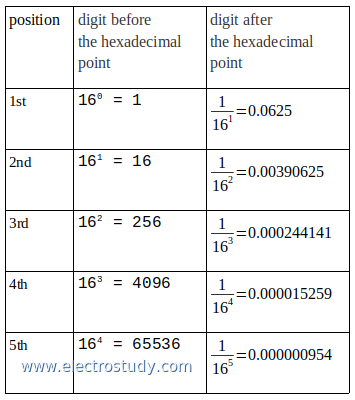# Hexadecimal fractions to decimal numbers

This post is explain how to convert hexadecimal fractions to decimal numbers. As we known, hexadecimal number is system number who have 16 digit numbers, which is 0, 1, 2, 3, 4, 5, 6, 7, 8, 9, A, B, C, D, E and F, where:
```A present of 10 B present of 11 C present of 12 D present of 13 E present of 14 F present of 15.```
The picture below is show hexadecimal fraction to decimal, form 1st position to 5th position of the digit, before and after the hexadecimal point.Hexadecimal fractions to decimal
Example 1:
Convert hexadecimal number 0.59A to decimal number

Completion:
0.59A (16) = ... (10)
1st digit after hexadecimal point: 5 → 5 × (1/ 161) = 5 × 0.0625 = 0.3125
2nd digit after hexadecimal point: 9 → 9 × (1/ 162) = 9 × 0.00390625 = 0.03515625
3rd digit after hexadecimal point: A → 10 × (1/ 163) = 10 × 0.000244141 = 0.00244141
sum all → 0.3125 + 0.03515625 + 0.00244141 = 0.35009766

0.59A (16) = 0.35009766 (10)

Example 2:
Convert hexadecimal number E2.81AC to decimal number

Completion:
E2.81AC (16) = ... (10)

1st digit: 2 → 2 × 160 = 2 × 1 = 2
2nd digit: E → 14 × 161 = 14 × 16 = 224
sum all → 2 + 224 = 226

1st digit: 8 → 8 × (1/ 161) = 8 × 0.0625 = 0.5
2nd digit: 1 → 1 × (1/ 162) = 1 × 0.00390625 = 0.00390625
3rd digit: A → 10 × (1/ 163) = 10 × 0.000244141 = 0.00244141
4th digit: C → 12 × (1/ 164) = 12 × 0.000015259 = 0.000183108
sum all → 0.5 + 0.00390625 + 0.00244141 + 0.000183108 = 0.506530768

E2.81AC (16) = 226.506530768 (10)
Next→
←Prev